# Parallel Current Density

The parallel current density takes the form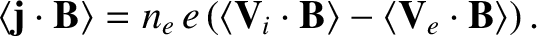(2.263)

It follows from Equations (2.221) and (2.257) that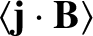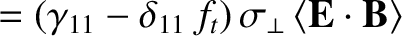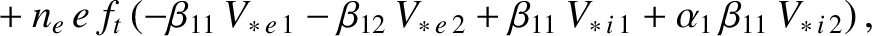(2.264)

where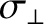is specified in Equation (2.41). In the circular magnetic flux-surface limit, the previous expression reduces to [30,52]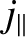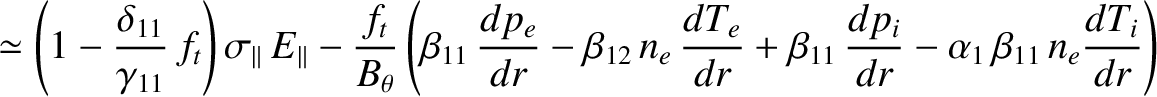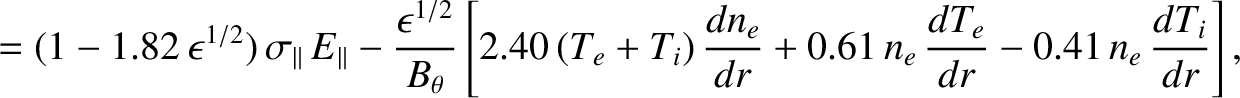(2.265)

where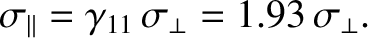(2.266)

Here, use has been made of Equations (2.202), (2.217), (2.243), (2.244), (2.247), and (2.251). Note that the previous formula agrees fairly well with the more accurate formula (2.40). (The reason for the slight discrepancy is that the moment-based expressions for the friction force densities given in Section 2.16 are only approximate.)

The first term on the right-hand side of Equation (2.265) represents the conventional ohmic parallel current driven by the inductive parallel electric field. Note, however, that the ohmic current is smaller than that predicted by the classical fluid closure scheme (see Section 2.6) by a factor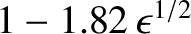. The explanation for this reduction is that trapped electrons cannot carry a net parallel current, because they are forced to periodically reverse their drift direction parallel to the magnetic field. Hence, the parallel electrical conductivity of the plasma is reduced by a factor that is roughly equal to the fraction of passing particles . [See Equation (2.201).] This effect is known as the neoclassical enhancement of (parallel) electrical resistivity, and has been observed in experiments [15,37,56].

The second term on the right-hand side of Equation (2.265) represents a non-inductive parallel current driven by equilibrium density and temperature gradients that is known as the bootstrap current . The bootstrap current has also been observed in experiments [54,55]. The ultimate origin of the bootstrap current is collisional friction between trapped and passing particles. It is, therefore, not surprising that the current is proportional to the fraction of trapped particles,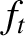.